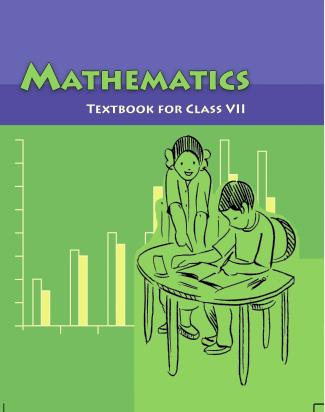1. /
2. CBSE
3. /
4. Class 07
5. /
6. Mathematics
7. /
8. NCERT Solutions for Class...

# NCERT Solutions for Class 7 Maths Exercise 2.6### myCBSEguide App

Download the app to get CBSE Sample Papers 2023-24, NCERT Solutions (Revised), Most Important Questions, Previous Year Question Bank, Mock Tests, and Detailed Notes.

NCERT solutions for Maths Fractions and Decimals## NCERT Solutions for Class 7 Maths Fractions and Decimals

###### Question 1.Find:

(i) 0.2 x 6

(ii) 8 x 4.6

(iii) 2.71 x 5

(iv) 20.1 x 4

(v) 0.05 x 7

(vi) 211.02 x 4

(vii) 2 x 0.86

(i) 0.2 x 6 = 1.2

(ii) 8 x 4.6 = 36.8

(iii) 2.71 x 5 = 13.55

(iv) 20.1 x 4 = 80.4

(v) 0.05 x 7 = 0.35

(vi) 211.02 x 4 = 844.08

(vii) 2 x 0.86 = 1.72

NCERT Solutions for Class 7 Maths Exercise 2.6

###### Question 2.Find the area of rectangle whose length is 5.7 cm and breadth is 3 cm.

Given: Length of rectangle = 5.7 cm and Breadth of rectangle = 3 cm

Area of rectangle = Length x Breadth

= 5.7 x 3 = 17.1{tex}c{m^2}{/tex}

Thus, the area of rectangle is 17.1 {tex}c{m^2}{/tex}.

NCERT Solutions for Class 7 Maths Exercise 2.6

###### Question 3.Find:

(i) 1.3 x 10

(ii) 36.8 x 10

(iii) 153.7 x 10

(iv) 168.07 x 10

(v) 31.1 x 100

(vi) 156.1 x 100

(vii) 3.62 x 100

(viii) 3.07 x 100

(ix) 0.5 x 10

(x) 0.08 x 10

(xi) 0.9 x 100

(xii) 0.03 x 1000

(i) 1.3 x 10 = 13.0

(ii) 36.8 x 10 = 368.0

(iii) 153.7 x 10 = 1537.0

(iv) 168.07 x 10 = 1680.7

(v) 31.1 x 100 = 3110.0

(vi) 156.1 x 100 = 15610.0

(vii) 3.62 x 100 = 362.0

(viii) 43.07 x 100 = 4307.0

(ix) 0.5 x 10 = 5.0

(x) 0.08 x 10 = 0.80

(xi) 0.9 x 100 = 90.0

(xii) 0.03 x 1000 = 30.0

NCERT Solutions for Class 7 Maths Exercise 2.6

###### Question 4.A two-wheeler covers a distance of 55.3 km in one litre of petrol. How much distance will it cover in 10 litres of petrol?

{tex}\because {/tex} In one litre, a two-wheeler covers a distance = 55.3 km

{tex}\therefore {/tex} In 10 litrs, a two- wheeler covers a distance = 55.3 x 10 = 553.0 km

Thus, 553 km distance will be covered by it in 10 litres of petrol.

NCERT Solutions for Class 7 Maths Exercise 2.6

###### Question 5.Find:

(i) 2.5 x 0.3

(ii) 0.1 x 51.7

(iii) 0.2 x 316.8

(iv) 1.3 x 3.1

(v) 0.5 x 0.05

(vi) 11.2 x 0.15

(vii) 1.07 x 0.02

(viii) 10.05 x 1.05

(ix) 101.01 x 0.01

(x) 100.01 x 1.1

(i) 2.5 x 0.3 = 0.75

(ii) 0.1 x 51.7 = 5.17

(iii) 0.2 x 316.8 = 63.36

(iv) 1.3 x 3.1 = 4.03

(v) 0.5 x 0.05 = 0.025

(vi) 11.2 x 0.15 = 1.680

(vii) 1.07 x 0.02 = 0.0214

(viii) 10.05 x 1.05 = 10.5525

(ix) 101.01 x 0.01 = 1.0101

(x) 100.01 x 1.1 = 110.11

## NCERT Solutions for Class 7 Maths Exercise 2.6

NCERT Solutions Class 7 Maths PDF (Download) Free from myCBSEguide app and myCBSEguide website. Ncert solution class 7 Maths includes text book solutions from Class 7 Maths Book . NCERT Solutions for CBSE Class 7 Maths have total 15 chapters. 7 Maths NCERT Solutions in PDF for free Download on our website. Ncert Maths class 7 solutions PDF and Maths ncert class 7 PDF solutions with latest modifications and as per the latest CBSE syllabus are only available in myCBSEguide.

## CBSE app for Students

To download NCERT Solutions for Class 7 Maths, Social Science Computer Science, Home Science, Hindi English, Maths Science do check myCBSEguide app or website. myCBSEguide provides sample papers with solution, test papers for chapter-wise practice, NCERT solutions, NCERT Exemplar solutions, quick revision notes for ready reference, CBSE guess papers and CBSE important question papers. Sample Paper all are made available through the best app for CBSE students and myCBSEguide website.### Test Generator

Create question paper PDF and online tests with your own name & logo in minutes.### myCBSEguide

Question Bank, Mock Tests, Exam Papers, NCERT Solutions, Sample Papers, Notes

### 2 thoughts on “NCERT Solutions for Class 7 Maths Exercise 2.6”

1. Thanks full of you

2. Please solution 2.5 and 2.7???